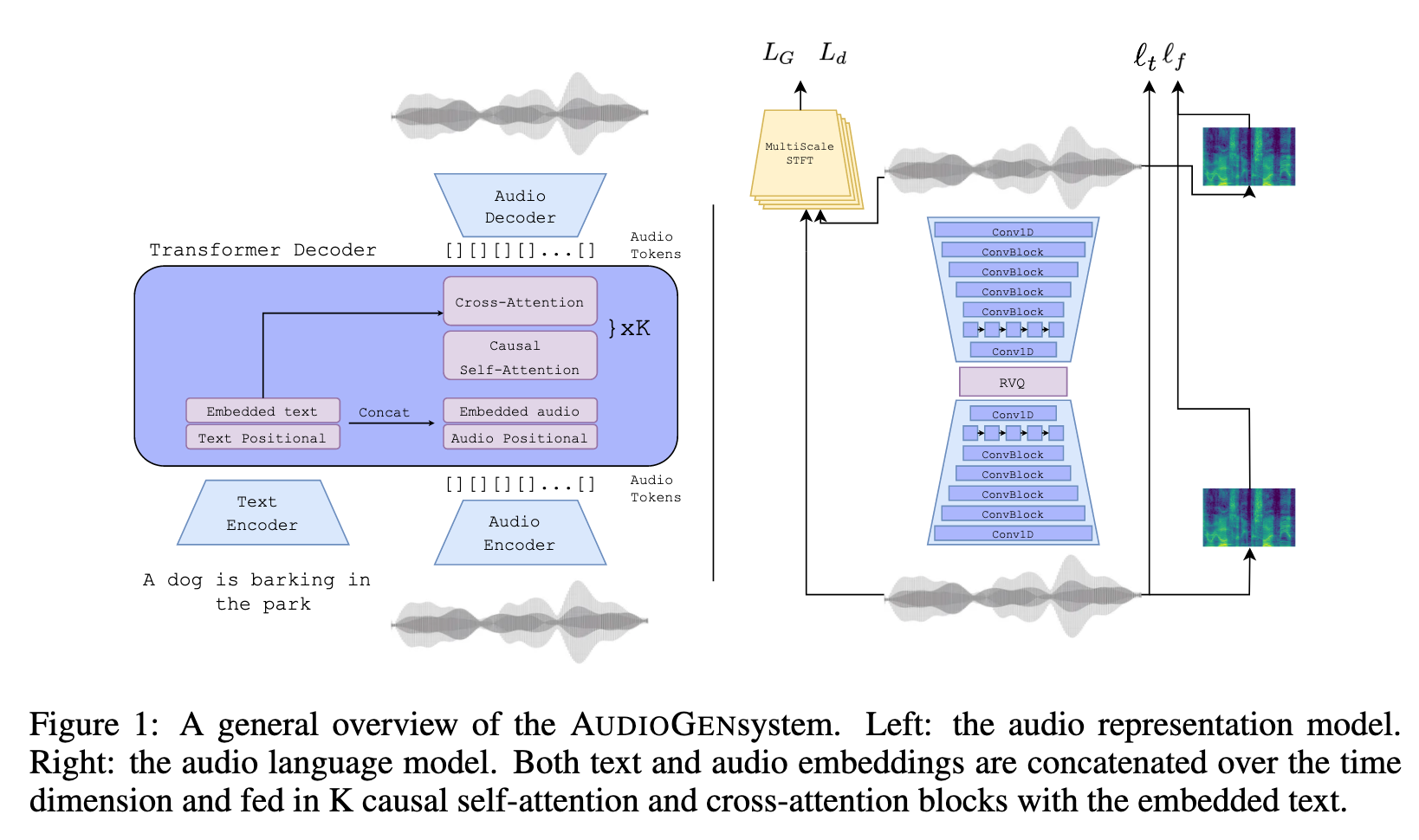# AudioGen Textually Guided Audio Generation

This is my reading note for AudioGen: Textually Guided Audio Generation. This paper propose to use auto regressive model to generate audio condition on text. The audio presentation is based on sound stream on neural sound.

# Introduction

we propose AUDIOGEN, an auto-regressive generative model that generates audio samples conditioned on text inputs. AUDIOGEN operates on a learnt discrete audio representation. To alleviate the aforementioned challenges we propose an augmentation technique that mixes different audio samples, driving the model to internally learn to separate multiple sources. For faster inference, we explore the use of multi-stream modeling, allowing the use of shorter sequences while maintaining a similar bitrate and perceptual quality (p. 1)

We propose AUDIOGEN, an autoregressive textually guided audio generation model. AUDIOGEN consists of two main stages. The first encodes raw audio to a discrete sequence of tokens using a neural audio compression model. The second stage, leverages an autoregressive Transformer-decoder language-model that operates on the discrete audio tokens obtained from the first stage while also being conditioned on textual inputs (p. 2)

# RELATED WORK

## Speech Representation Learning

van den Oord et al. (2018) and Schneider et al. (2019) suggested training a convolutional neural network to distinguish true future samples from random distractor samples using a Contrastive Predictive Coding (CPC) loss function. HuBERT model which is trained with a masked prediction task similar to BERT. Recent studies suggest quantizing SSL representations using k-means and later perform language modeling.

## Text-to-Audio

DiffSound, a text-to-audio model based on a diffusion process that operates on audio discrete codes. The audio codes were obtained from a VQ-VAE (van den Oord et al., 2017a) based model trained over mel-spectrogram. They additionally explore the usage of an auto-regressive Transformer decoder model, however found it to be inferior to the diffusion based model. (p. 3)

The proposed method differentiate from DiffSound in the following: (i) our audio representation is being learned directly from the raw-waveform; (ii) we create new audio compositions using data augmentation allowing the model to generate audio from complex text captions; (iii) we apply and study the effect of classifier free guidance under the auto-regressive setting; (iv) in contrast to Yang et al. (2022), we empirically demonstrate that text-conditioned auto-regressive models can generate high-quality audio samples. (p. 3)

# Method

## AUDIO REPRESENTATION

For Q, we use a single codebook with 2048 codes, where each code is a 128 dimensional vector (p. 3)We use ELU as a non-linear activation function (Clevert et al., 2015) and a LayerNorm (Ba et al., 2016). (p. 4)

We optimize a GAN based training objective similar to (Kong et al., 2020; Zeghidour et al., 2021) of jointly minimizing a combination of reconstruction losses and adversarial losses. Specifically, we minimize the L1 distance between the target and reconstructed audio over the time domain, i.e. $l_t(x, \hat{x}) = \lVert x−\hat{x}\rVert_1$. For the frequency domain loss, we use a linear combination between the L1 and L2 losses over the mel-spectrogram using several time scales (p. 4)

## AUDIO LANGUAGE MODELING

Although the CFG method was originally proposed for the score function estimates of diffusion models, in this work we apply it to auto-regressive models. During training we optimize the Transformer-LM conditionally and unconditionally. In practice, we randomly omit the text conditioning in 10% of training samples. At inference time we sample from a distribution obtained by a linear combination of the conditional and unconditional probabilities (p. 5)

Written on July 24, 2023